# Father and son

Father and son weigh together 108kg. The father weighs 2.6 times more than his son. How much does weight my father and son count?

a =  78
b =  30

### Step-by-step explanation:

a+b=108
a = 2.6 b

a+b=108
a = 2.6•b

a+b = 108
a-2.6b = 0

a = 78
b = 30

Our linear equations calculator calculates it.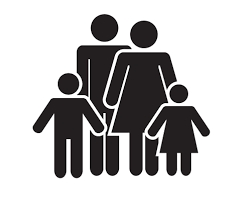Did you find an error or inaccuracy? Feel free to write us. Thank you!Tips to related online calculators
Do you have a linear equation or system of equations and looking for its solution? Or do you have a quadratic equation?

## Related math problems and questions:

• HomeworkMother and Kate together weigh 24.5 kg more than the father. Father weighs 7.6 kg more than mother. Father weight 44.8 kg more than Kate. Determine weights of father mother and Kate.
• The devils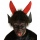The devils weighed in hell with Dorota. They found that Dorota and the two devils weigh 250 kg together, and Dorota and the four devils weigh 426 kg. All the devils weigh the same. How Much Does Dorota Weigh?
• Children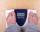Margaret and Zdena weigh the same and Petra 3 kg more. Together weigh 156 kg. How much do they weigh?
• BucketThe bucket half filled with water weighs 5.55 kg, the full bucket weighs 9.85 kg. How much does the bucket weigh?
• Two melons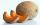There are 2 melons in the box. Together they weigh 11kg. The weight of green is 3 kg less than three times the weight of yellow. What is the weight of a green melon?
• Six hensSix hens weigh 3 kg more than three geese and 7 kg less than five geese. How much does a hen weigh, and how many geese?
• Trio weight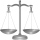Adelka, Barunka, and Cecilka are weight in pairs. Adelka with Barunka weighs 98 kg, Barunka with Cecilka 90 kg and Adelka with Cecilka 92 kg. How much does each of them weigh?
• Empty canisterThe canister is filled with oil weight 17 kg. If filled only half weight 9 kg. What weight does my empty canister have?
• Shopping vegetablesJohn and his mother were shopping. My mother bought 2 kg of peaches and 5 kg of potatoes and paid CZK 173. The neighbor bought 3 kg of peaches and 4 kg of potatoes and paid CZK 186. How much did 1 kg of peaches and 1 kg of potatoes cost?
• Barrel 4Barrel of water weighs 63 kg. After off 75% water, the weight of the barrel with water is 21 kg. How many kg weigh empty barrel and how many kgs water in it?
• ApplesApples I picked 600 kg this year. Autumn's apples were four times more than the summer. How many kg's I picked in the summer and how many winter apples?
• Watering can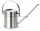A watering can full of water weighs 11 kg, the weight of water is 10 kg greater than the weight of an empty watering can, how much is the weight of the watering can?
• Three friendsDanica, Lenka, and Dalibor have 96 kg altogether. Lenka weighs 75% more than Dalibor, and Danica weighs 6 kg more than Dalibor. Determine the weight of Danice, Lenka, and Dalibor.
• Father and son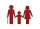When I was eight years old, my father was 30 years old. Today, my father is three times more than me. How old am I?
• Father and son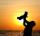Father is three times older than his son. Twelve years ago, the father was nine times more senior than the son. How old are father and son?
• Bent scaleMonica weighed 52 kg. Sara 54 kg. Together they weighed 111 kg. They noticed that the weight on the scale was bent. How much did they really weigh?
• Apples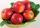Zuzana bought 3 kg Jonathan apples. Then she noticed that Golden apples were 3 CZK a kilogram cheaper. So they bought 2 kg. For apples, she paid a total of CZK 109. How much did 1 kg Jonathan and how much Golden?